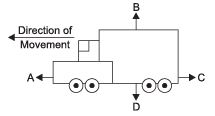Courses

# Very Short Answers - Force and Laws of Motion, Science, Class 9 Class 9 Notes | EduRev

## Class 9 : Very Short Answers - Force and Laws of Motion, Science, Class 9 Class 9 Notes | EduRev

The document Very Short Answers - Force and Laws of Motion, Science, Class 9 Class 9 Notes | EduRev is a part of the Class 9 Course Class 9 Science by VP Classes.
All you need of Class 9 at this link: Class 9

Q1. Define force.
Ans.
It is a push or pull on an object that produces acceleration in the body on which it acts.

Q2. What is S.I. unit of force?
Ans.
S.I. unit of force is Newton.

Q3. Define one Newton.
Ans.
A force of one Newton produces an acceleration of 1 m/s2 on an object of mass 1 kg. 1 N = 1 kg m/s2

Q4. What is balanced force?
Ans.
When forces acting on a body from the opposite direction do not change the state of rest or of motion of an object, such forces are called balanced forces.

Q5. What is frictional force?
Ans.
The force that always opposes the motion of an object is called force of friction.

Q6. What is inertia?
Ans.
The natural tendency of an object to resist a change in their state of rest or of uniform motion is called inertia.

Q7. State Newton’s first law of motion.
Ans.
An object remains in a state of rest or of uniform motion in a straight line unless acted upon by an external unbalanced force.

Q8. State Newton’s second law of motion.
Ans.
The rate of change of momentum of an object is proportional to the applied unbalanced force in the direction of the force.

Q9. What is momentum?
Ans.
The momentum of an object is the product of its mass and velocity and has the same direction as that of the velocity. The S.I. unit is kg m/s. (p = mv)

Q10. State Newton’s III law of motion.
Ans.
To every action, there is an equal and opposite reaction and they act on two different bodies.

Q11. Which will have more inertia a body of mass 10 kg or a body of mass 20 kg?
Ans.
A body of mass 20 kg will have more inertia.

Q12. Name the factor on which the inertia of the body depends.
Ans
. Inertia of a body depends upon the mass of the body.

Q13. Name two factors which determine the momentum of a body.
Ans.
Two factors on which momentum of a body depend is mass and velocity.
Momentum is directly proportional to the mass and velocity of the body.

Q14. What decides the rate of change of momentum of an object?
Ans.
The rate of change of momentum of an object is proportional to the applied unbalanced force in the direction of force.

Q15. The diagram shows a moving truck. Forces A, B, C and D are acting on the truck.
Name the type of forces acting on a truck.
Ans.
The forces A, B, C and D acting on the truck are:. The forces A, B, C and D acting on the truck are:
A  → driving force
B  → reacting force
C  → frictional force
D  → weight/gravitational force

Q16. A 68 kg runner exerts a force of 59 N. What is the acceleration of the runner?
Ans.
m = 68 kg, F = 59N, a = ?

F= ma,

59 = 68a

a= 59/68 = 0.87 m/s2

Q17. A trolley is dragged across an ice covered lake. The box accelerates at 0.08 m/s2 and is pulled by a 47 N force. What is the mass of the box?
Ans.
a = 0.08 m/s2, F = 47N, m = ?

F=ma,

47 = m(0.08)

m= 47/0..8 = 587.5 kg

Q18. A tennis ball, 0.314 kg, is accelerated at a rate of 164 m/s2 when hit by a professional tennis player. What force does the player’s tennis racket exert on the ball?
Ans.
m = 0.314 kg, a = 164 m/s2,
F = ?
F = ma = (0.314)(164) = 51.50 N

Q19. A coolie exerts a force of 628 N on a luggage trolley. Later, the mass of the trolley is increased by a factor of 3.8. If the coolie exerts the same force, how does the new acceleration compare to the old one?
Ans.
a = (F/m)
⇒   a = 628N/3.8
= 165.26 N

Q20. What is the mass of a runner whose weight is 648 N?
Ans
. g = 9.80 m/s2,

W=mg

m= 66.12 kg

= m= W/g

= m=648/9.8

Q21. When you jump, the Earth is pushed away from you. Use a formula to explain why this movement of the Earth isn’t noticed.
Ans.
a = (F/m) the Earth has lot of mass that its acceleration is tiny.

Q22. Compare the force on a cricket ball by a bat with the force exerted by the ball on the bat.
Ans.
The force has the same magnitude but opposite direction.

Q23. A cart is being moved by a certain net force. If more force is applied on the cart, doubling its mass, state by how much the acceleration changes.
Ans
. It will be half.

Q24. A hammer is hitting a nail. Compare the force on the nail; with the force on the hammer.
Ans.
The forces acting on nail and on hammer are equal in magnitude (strength) but opposite in direction.

Offer running on EduRev: Apply code STAYHOME200 to get INR 200 off on our premium plan EduRev Infinity!

75 docs|14 tests

,

,

,

,

,

,

,

,

,

,

,

,

,

,

,

,

,

,

,

,

,

,

,

,

,

,

,

;